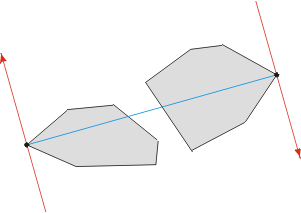• 如果个坐标的列(x1,y1)、(x2,y2)，那么他们之间的距离：SQRT((X1-X2)(X1-X2)+(Y1-Y2)(Y1-Y2)) 例：/* 表名：TpProject FLongitude：经度 FDimension：纬度 传入的参数：（121.517759,31.178469） */ ...
如果两个坐标的列是(x1,y1)、(x2,y2)，那么他们之间的距离：SQRT((X1-X2)(X1-X2)+(Y1-Y2)(Y1-Y2))
例：

/*
表名：TpProject
FLongitude：经度
FDimension：纬度
传入的参数：（121.517759,31.178469）
*/
SELECT * FROM TpProject
ORDER BY
SQRT((121.517759-FLongitude)*(121.517759-FLongitude)+(31.178469-FDimension)*(31.178469-FDimension))  
展开全文sql
• 题意：有一群牛，若其中两头牛一起看过一场电影，那么它们关系1度，如果两头牛没有看过同一场电影但它们都与第三头牛1度关系，那么他们的关系2度。保证没两头牛之间都有关系，求与其他牛之间关系平均...
题意：有一群牛，若其中两头牛一起看过一场电影，那么它们的关系是1度的，如果两头牛没有看过同一场电影但它们都与第三头牛是1度的关系，那么他们的关系是2度的。保证没两头牛之间都有关系，求与其他牛之间关系平均度数最小的牛的 平均度数*100，即先找出与其他牛关系度数和的最小。
思路：用floyd（），得到没头牛与其他牛关系度数的最小值。

#include
#include
int together, degree;
int n;
void floyd()
{
for(int k = 1; k <= n; k++)
for(int i = 1; i <= n; i++)
for(int j = 1; j <= n; j++)
{
if(degree[k][i] && degree[k][j])
{
if(degree[i][j] == 0
|| degree[i][j] > degree[k][i] + degree[k][j])
{
degree[i][j] = degree[k][i] + degree[k][j];
}
}
}
}

int main()
{
#ifdef LOCAL
freopen("data.in", "r", stdin);
#endif

int m, num;
double sum, ans; // 最后度数之和要除以n-1，要保留小数点后两位
while(scanf("%d%d", &n, &m) != EOF)
{
//printf("n=%d,m=%d\n", n, m);
memset(degree, 0, sizeof(degree));
while(m--)
{
scanf("%d", &num); //printf("m=%d, num=%d\n", m, n);
for(int i = 0; i < num; i++)
{
scanf("%d", &together[i]);
for(int j = 0; j < i ; j++)
{

degree[together[i]][together[j]]
= degree[together[j]][together[i]] = 1;
}
}

}
floyd();
ans = 100000000.0;
for(int i = 1; i <= n; i++)
{
sum = 0;
for(int j = 1; j <= n; j++)
{
if(i != j)
{
sum += degree[i][j] * 1.0;
}
}
if(sum < ans) ans = sum;
}
printf("%d\n", (int)(ans / (n - 1) * 100));
}
return 0;
}



展开全文• 求距离最近的他们之间的距离。 分治法： 将一个大问题缩减到一定的规模变成若干个相同的小问题一一解决。它与动态规划法类似，都将问题分而治之，变成若干个能够解决小问题，但是动态规划一般分解问题后...
平面上有n个点P1,P2,…,Pn，n>1,Pi的直角坐标为(Xi,Yi),i=1,2,…,n.求距离最近的两个点及他们之间的距离。
分治法：
将一个大问题缩减到一定的规模变成若干个相同的小问题一一解决。它与动态规划法类似，都是将问题分而治之，变成若干个能够解决小问题，但是动态规划一般分解问题后问题间会互相连续和依赖，而分治法分解后变成一个个独立的问题。
思路
1.在一个平面内的点，先将他们按照x坐标进行排序，将它们以x的中间值的纵轴划分为左右两部分，分别递归找到这两部分中两点之间的最短距离，然后求出左边部分的一个点和右边部分一个点之间最短距离，他们的最小值既是最短距离的两个点。
package algorithm;

import java.util.Arrays;

/**
* @author Tcc
*/
public class ShortestDistance {

private double[][] points;

Point p1, p2;

public ShortestDistance(double[][] points) {
this.points = points;
}

public double getMinimumDistance() {
Point[] orderByx = new Point[points.length];
for (int i = 0; i < orderByx.length; i++){
orderByx[i] = new Point(points[i], points[i]);
}
//按照x坐标排序
Arrays.sort(orderByx);
// 重复点距离为0，不符合题意
if (checkIdentical(orderByx)) {
return 0;
}
Point[] orderByy = orderByx.clone();
//按照y坐标排序，方便后续比较距离时简单点
Arrays.sort(orderByy, new CompareY());
return distance(orderByx, 0,orderByx.length - 1, orderByy);
}

/**
* 检查重复点
*/
public boolean checkIdentical(Point[] orderByx) {
for (int i = 0; i < orderByx.length - 1; i++) {
if (orderByx[i].compareTo(orderByx[i + 1]) == 0) {
p1 = orderByx[i];
p2 = orderByx[i + 1];
return true;
}
}
return false;
}

/**
* 返回最近的距离
*/
public double distance( Point[] orderByx, int low, int high,Point[] orderByy) {

//两个点以下的情况
if (low >= high){
return Double.MAX_VALUE;
} else if (low + 1 == high) {
p1 = orderByx[low];
p2 = orderByx[high];
return distance(orderByx[low], orderByx[high]);
}

//把X分为两组，左边和右边
int mid = (low + high) / 2;
Point[] orderByyLeft = new Point[mid - low + 1];
Point[] orderByyRight = new Point[high - mid];
int j1 = 0;
int j2 = 0;
for (int i = 0; i < orderByy.length; i++) {
if (orderByy[i].compareTo(orderByx[mid]) <= 0){
orderByyLeft[j1++] = orderByy[i];
} else{
orderByyRight[j2++] = orderByy[i];
}
}
//递归找到左边和右边的最近距离值
double dLeft = distance(orderByx, low, mid, orderByyLeft);
double dRight = distance(orderByx, mid + 1, high, orderByyRight);
double d = Math.min(dLeft, dRight);

// 找到左边离中线距离小于最小距离d的点
int count = 0;
for (Point orderByyLeftz : orderByyLeft) {
if (orderByyLeftz.x >= orderByx[mid].x - d){
count++;
}
}
Point[] leftPoint = new Point[count];
count = 0;
for (Point orderByyLeftz : orderByyLeft) {
if (orderByyLeftz.x >= orderByx[mid].x - d){
leftPoint[count++] = orderByyLeftz;
}
}

// 找到右边离中线距离小于最小距离d的点
count = 0;
for (Point orderByyRightz : orderByyRight) {
if (orderByyRightz.x <= orderByx[mid].x + d){
count++;
}
}
Point[] rightPoint = new Point[count];
count = 0;
for (Point orderByyRightz : orderByyRight) {
if (orderByyRightz.x <= orderByx[mid].x + d){
rightPoint[count++] = orderByyRightz;
}
}

// 找到这些小于d的点中 左边和右边两边距离最小的值
double dMid = d;
int j = 0;
for (Point aLeftPoint : leftPoint) {
//过滤一些值
while (j < rightPoint.length && aLeftPoint.y > rightPoint[j].y + d) {
j++;
}
int k = j;
while (k < rightPoint.length && rightPoint[k].y <= aLeftPoint.y + d) {
if (dMid > distance(aLeftPoint, rightPoint[k])) {
dMid = distance(aLeftPoint, rightPoint[k]);
p1 = aLeftPoint;
p2 = rightPoint[k];
}
k++;
}
}
return Math.min(d, dMid);
}

/**
* 计算p1和p2的坐标
*/
public static double distance(Point p1, Point p2) {
return Math.sqrt(Math.pow(p2.x-p1.x,2)+Math.pow((p2.y-p1.y),2));
}

/**
* 定义一个有x和y坐标点的类
*/
static class Point implements Comparable<Point> {
double x;
double y;
Point(double x, double y) {
this.x = x;
this.y = y;
}
@Override
public int compareTo(Point p2) {
if (this.x < p2.x) {
return -1;
} else if (this.x == p2.x) {
return Double.compare(this.y, p2.y);
} else {
return 1;
}
}

}

/**
* 先比较y坐标，y坐标相同比较x坐标
*/
static class CompareY implements java.util.Comparator<Point> {
@Override
public int compare(Point p1, Point p2) {
if (p1.y < p2.y) {
return -1;
} else if (p1.y == p2.y) {
return Double.compare(p1.x, p2.x);
} else{
return 1;
}
}
}

public static void main(String[] args) {

//随机生成30个点
double[][] points = new double;
for (int i = 0; i < points.length; i++) {
points[i] = Math.random() * 10;
points[i] = Math.random() * 10;
}
ShortestDistance shortestDistance = new ShortestDistance(points);
System.out.println("最短距离：" + shortestDistance.getMinimumDistance());
System.out.print("(" + shortestDistance.p1.x + ", " + shortestDistance.p1.y + ")和"+"(" + shortestDistance.p2.x + ", " + shortestDistance.p2.y + ")");
System.out.println();
}
}



展开全文java
• 给定个凸多边形 P 和 Q， 目标需要找到对 (p,q) （p 属于 P 且 q 属于 Q） 使得他们之间的距离最大。  很直观地，这些不可能属于他们各自多边形的内部。 这个条件事实上与直径问题非常相似： ...


给定两个凸多边形 P 和 Q， 目标是需要找到点对 (p,q) （p 属于 P 且 q 属于 Q） 使得他们之间的距离最大。
很直观地，这些点不可能属于他们各自多边形的内部。 这个条件事实上与直径问题非常相似：
两凸多边形 P 和 Q 间最大距离由多边形间的对踵点对确定。
虽然说法一样， 但是这个定义与给定凸多边形的对踵点对的不同。
与凸多边形间的对踵点对本质上的区别在于切线是有向且反向的。 下图展示了一个例子：上述结论暗示不单纯只是顶点对需要检测， 而仅仅是特定的顶点对需要被考虑到。 事实上他们只检测一个基于旋转卡壳模式的算法确立的平行切线。
考虑如下的算法， 算法的输入是两个分别有 m 和 n 个顺时针给定顶点的凸多边形 P 和 Q。
计算 P 上 y 坐标值最小的顶点（称为 yminP ） 和 Q 上 y 坐标值最大的顶点（称为 ymaxQ）。为多边形在 yminP 和 ymaxQ 处构造两条切线 LP 和 LQ 使得他们对应的多边形位于他们的右侧。 此时 LP和 LQ 拥有不同的方向， 并且 yminP 和 ymaxQ 成为了多边形间的一个对踵点对。计算距离(yminP,ymaxQ) 并且将其维护为当前最大值。顺时针同时旋转平行线直到其中一个与其所在的多边形的边重合。一个新的对踵点对产生了。 计算新距离， 与当前最大值比较， 如果大于当前最大值则更新。 如果两条线同时与边发生重合， 此时总共三个对踵点对（先前顶点和新顶点的组合）需要考虑在内。重复执行步骤4和步骤5， 直到新的点对为(yminP,ymaxQ)。输出最大距离。
旋转卡壳模式确保了所有的对踵点对都被考虑到。 此外， 整个算法拥有线性的时间复杂度， 因为（除了初始化）， 执行步数与顶点数相同。
类似的算法可以被用于凸多边形间最小距离问题中。

原文地址：http://cgm.cs.mcgill.ca/~orm/maxd2p.html


展开全文• 给定个凸多边形 P 和 Q， 目标需要找到对 (p,q) （p 属于 P 且 q 属于 Q） 使得他们之间的距离最大。 很直观地，这些不可能属于他们各自多边形的内部。 这个条件事实上与直径问题非常相似：  ...
• 这边 我的解法 利用了一个很重要的性质---树的直径--树上任意两点间的最远距离 而同时 将这个直径上的两点x , y确定后 那么 整个树上结点的dist[ i ]也就可以确定了 max( dist[i][x] , dist[i][y] ) 要么到x...
• 欧几里德距离指多维空间两点间的距离，这一种用直尺测量出来的距离。如果将两个点分别标记为(p1,p2,p3....pn)和(q1,q2,q3.....qn),则欧几里德距离的计算公式为：     欧几里德距离评价算法一个...
• 工作中要跟据GPS得到经纬度计算两点间距离，在网上找了几个公式和程序想对比，下面这个好像比较专业，但里面有几个问题请高手指点啊，急啊，拜托了问题1：里面用e取值多少啊，科学计数中10，自然数2.718282...算法 float
• 乘坐出租车从家到公司，下车后计价表显示30公里，这可不是两点间的直线。《三国》里，探马回报：“袁军距我军30里处的官渡处下寨，绵延百里”,到底30里还是百里，怎样才算30里？2018年法国队赢得世界杯冠军，距离...
• 给定个凸多边形 P 和 Q， 目标需要找到对 (p,q) （p 属于 P 且 q 属于 Q） 使得他们之间的距离最大。  很直观地，这些不可能属于他们各自多边形的内部。 这种情况事实上与直径问题非常相似：  ...算法
• 我们再假设，环入口在点q，从头节点到点q距离为A，q p两点间距离为B，p q两点间距离为C。 因为快指针慢指针两倍速，且他们在p点相遇，则我们可以得到等式 2(A+B) = A+B+C+B. （此处应为：如果环前
• 欧几里德距离指多维空间两点间的距离，这一种用直尺测量出来的距离。如果将两个点分别标记为(p1,p2,p3....pn)和(q1,q2,q3.....qn),则欧几里德距离的计算公式为：     欧几里德距离评价算法一个非常简单的...python 欧几里德 数据挖掘 数据分析 算法
• 先想想暴力做法吧，n3的，都会吧，暴力枚举两点，再暴力求出他们间的距离，判断是否小于k，统计答案。 n2log呢？很简单吧，就是把暴力求距离那一步改成LCA嘛。 那么我们的点分治就更加牛逼了，它可以在n log2 的...
• 而对于这道题来说求出任意两点间的距离是不现实的，但是利用异或的性质，如果我们有了每个点到根的距离，就能间接得到任意两点间的距离，对于任意两点u和v，他们之间的距离就是他们到根的距离异或起来。因为不在u到v...
• Mathematica model ;主要内容;图的模型;图论的起源七桥问题;一般用大写字母G,H表示无向图; ? e与顶点u, v相关联 ? u与v相邻 ?... 设S=x1,x2xn平面上的点集其中任意两点间的距离至少1证明距离正好1的点
• 然后给定你一个mmm，问你能找到mmm个黑色节点使得他们之间任意两点间的距离的最大值最下为多少，输出这个最小值。 输入保证一定存在答案，保证nnn个节点构成一棵树。 思路： 首先应该知道树的直径的定义： a. 树上...
• 题目描述: 给一个链表，若其中包含...我们再假设，环入口在点q，从头节点到点q距离为A，q p两点间距离为B，p q两点间距离为C。 因为快指针慢指针两倍速，且他们在p点相遇，则我们可以得到等式 2(A+B) = A+B+C+B.链表 指针 java 算法 数据结构
• 我们再假设，环入口在点q，从头节点到点q距离为A，q p两点间距离为B，p q两点间距离为C。 因为快指针慢指针两倍速，且他们在p点相遇，则我们可以得到等式 2(A+B) = A+B+C+B.（此处应为：如果环前面.
• 题目描述给你一棵树,两两节点间有一个距离cic_i,现在要重建地图，任意个顶点间的花费是他们之间的最短路,建最大生成树求花费.分析这题比赛的时候没有做出来,赛后看题解原来很简单,想象prim的建树过程每次找剩余边...prim
• 如果, 某几个城市两两都可以相互传递信息,那代表他们是一个国家. 输入E代表可以连通 城市有那几对,X->Y 耗时H. 一个国家之间传递信息可以瞬间完成. 所以这是一个有向有环图. 通过tarjan 缩. 去环后. 把每...强连通
• ## 两款定时提醒休息工具-IT人士必备

千次下载 热门讨论 2012-03-14 11:31:20
更糟糕的是，许多人一天坐着时间长达15小时，这意味着，他们连散步时间都花在沙发上，椅子上和车上了。 久坐并不是很难克服坏习惯，关键在个细节：日常活动、注意久坐时间。我们先来看看久坐怎样影响身体...
• 题意：有n个点，他们之间有n-1条无向边，形成一棵树，并且保证任意点间都不存在条不同的路径可以互相到达。求这棵树中哪个结点之间的距离最长？这里的距离是指从一个结点走到另一个结点经过的边数。 解析：...hihoCoder
• 给出k组点，求出组内两点间的最大距离 核心思路 考虑贪心，每组内的最深一点一定是两最远距离点对之一。 证明很简单，可以分为在该点的祖先相同和祖先不同的情况。如果点对其中一个为另一个的祖先的话，一定会选择...
• 凸多边形的宽度定义为平行切线间的最小距离。 这个定义从宽度这个词中已经略有体现。 虽然凸多边形的切线有... 通过绕着这旋转这条线， 使他们之间的距离递增或递减。 特别的， 总存在一个 特定旋转方向 使得
• 由于经费问题，他们想在任意两点间的距离最短的前提下，用尽可能少的投资把各个点连接起来。需要注意的并不是任意两个居民点间都能直接相连给出两两居民点间建路的花费（与长度成正比），你可以帮他们选择一个最佳...
• 题目描述抽象来看，指...有一个例子说求两点间经过N条边到达方案数..Mtrix67Blog第八题讲这个问题.... 首先看经过N条边方案数这个问题...也就是理解一下这个过程...用一个邻接矩阵来存图...点 ...